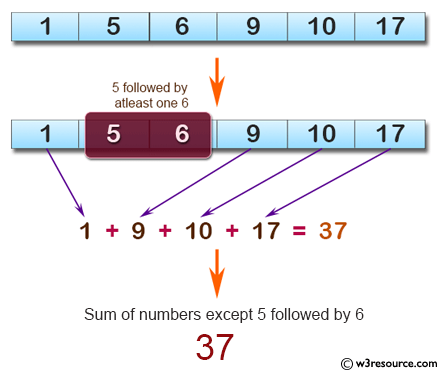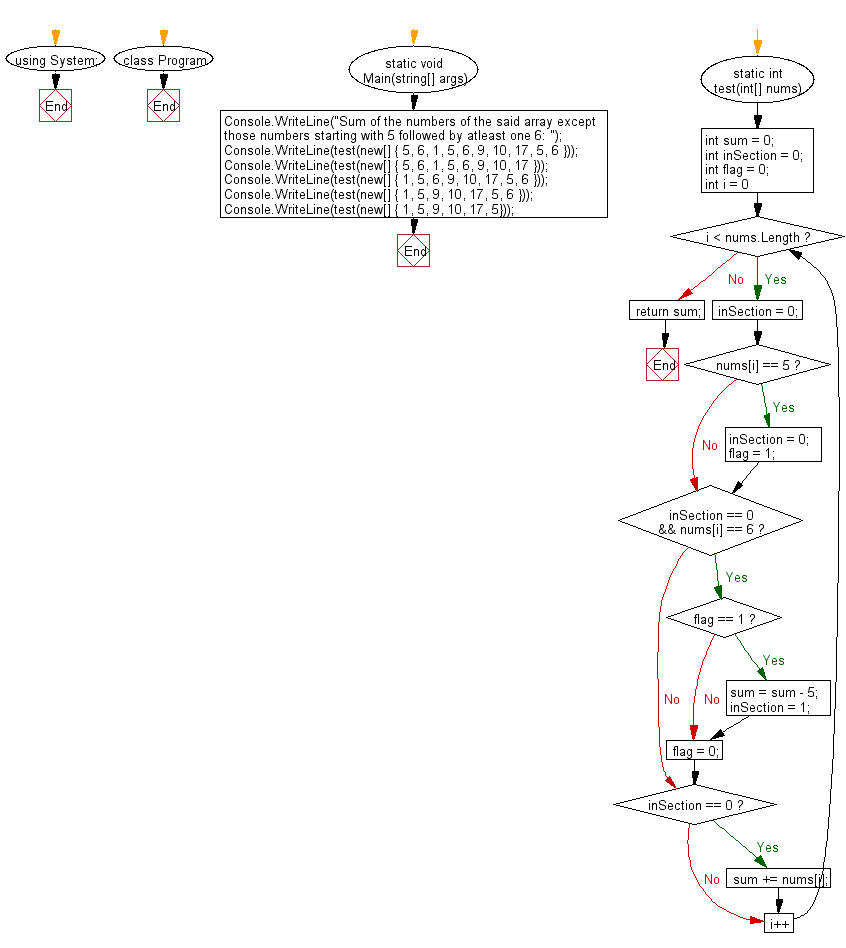﻿ C# - Sum numbers in an array except start with 5, end with 6# C# Sharp Basic Algorithm Exercises: Compute the sum of the numbers in a given array except those numbers starting with 5 followed by at least one 6

## C# Sharp Basic Algorithm: Exercise-112 with Solution

Write a C# Sharp program to compute the sum of the numbers in a given array except those numbers starting with 5 followed by at least one 6. Return 0 if the given array has no integer.

Pictorial Presentation:Sample Solution:-

C# Sharp Code:

``````using System;
namespace exercises
{
class Program
{
static void Main(string[] args)
{
Console.WriteLine("Sum of the numbers of the said array except those numbers starting with 5 followed by at least one 6: ");
Console.WriteLine(test(new[] { 5, 6, 1, 5, 6, 9, 10, 17, 5, 6 }));
Console.WriteLine(test(new[] { 5, 6, 1, 5, 6, 9, 10, 17 }));
Console.WriteLine(test(new[] { 1, 5, 6, 9, 10, 17, 5, 6 }));
Console.WriteLine(test(new[] { 1, 5, 9, 10, 17, 5, 6 }));
Console.WriteLine(test(new[] { 1, 5, 9, 10, 17, 5}));
}
static int test(int[] nums)
{
int sum = 0;
int inSection = 0;
int flag = 0;
for (int i = 0; i < nums.Length; i++)
{
inSection = 0;
if (nums[i] == 5)
{
inSection = 0;
flag = 1;
}
if (inSection == 0 && nums[i] == 6)
{
if (flag == 1)
{
sum = sum - 5;
inSection = 1;
}
flag = 0;
}
if (inSection == 0)
{
sum += nums[i];
}
}
return sum;
}
}
}
```
```

Sample Output:

```Sum of the numbers of the said array except those numbers starting with 5 followed by at least one 6:
37
37
37
42
47```

Flowchart:C# Sharp Code Editor:

Improve this sample solution and post your code through Disqus

What is the difficulty level of this exercise?

Test your Programming skills with w3resource's quiz.

﻿